# HTML5 Canvas : Collision Detection

In 2D environment, the common collision detection methods are as follows:

### External figure discrimination

• Axisymmetric bounding box, i.e. rectangle without rotation.
• Circular collision
• Circle and rectangle (no rotation)
• Circle and rotation rectangle (with the center of the rectangle as the rotation axis)

### Separation axis theorem

Other people

• Map grid division
• Pixel detection

The following will introduce the above mentioned collision detection methods from easy to difficult order: external graph discrimination > other > ray casting > separation axis theorem.

In addition, there are some scenarios where we can achieve the collision we want as long as we have agreed on the limiting conditions, such as the following collision bounce:

See the Pen Boundary collision detection by Jc (@JChehe) on CodePen.

When the ball touches the border, it bounces (for example, the speed in X / Y direction is reversed).

``````if(ball.left < 0 || ball.right  > rect.width)  ball.velocityX = -ball.velocityX
if(ball.top  < 0 || ball.bottom > rect.height) ball.velocityY = -ball.velocityY
``````

For example, when a person goes to the 100px position without jumping, he will encounter stones and so on.

Therefore, some scenes only need to set appropriate parameters to achieve collision detection.

## External figure discrimination

### Axis aligned bounding box

Concept: judge whether any side of any two (non rotating) rectangles has no spacing, so as to judge whether they collide.

Algorithm:

``````rect1.x < rect2.x + rect2.width &&
rect1.x + rect1.width > rect2.x &&
rect1.y < rect2.y + rect2.height &&
rect1.height + rect1.y > rect2.y
``````

Various situations of collision between two rectangles: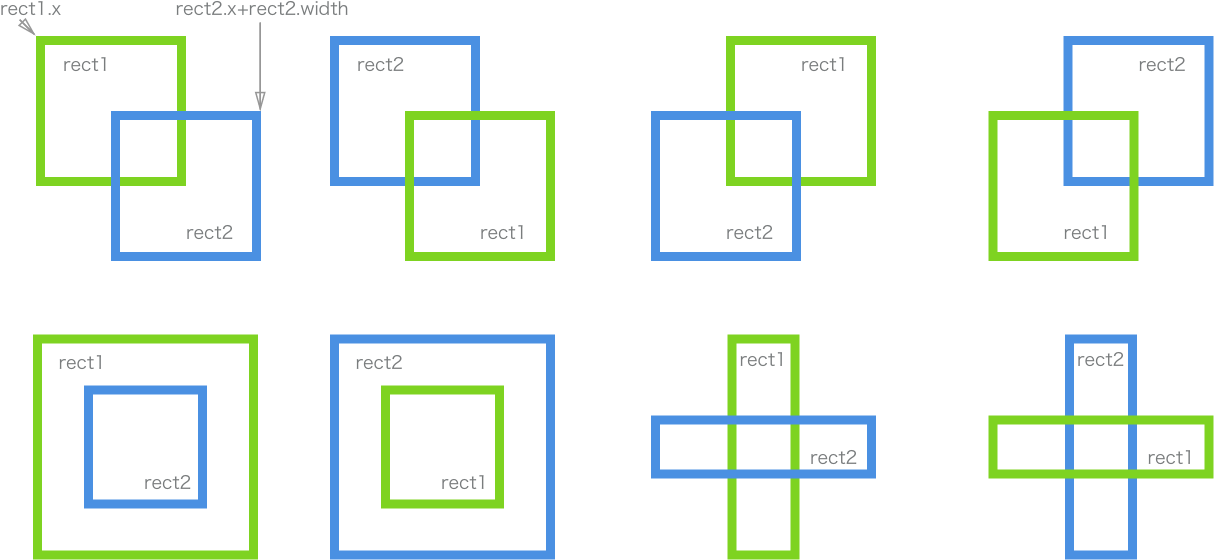Online operation example (first click on the operation example to get the focus, the same below):

See the Pen AxisAlignedBoundingBox collision detection by Jc (@JChehe) on CodePen.

• Relative limitation: the two objects must be rectangular, and neither of them is allowed to rotate (i.e. symmetrical with respect to horizontal and vertical directions).
• For collision detection of rectangles containing patterns (not filling the whole rectangle), there may be insufficient precision.
• When the moving speed of the object is too fast, it may cross between two adjacent animation frames quickly, resulting in the event that the collision is ignored.

Applicable cases:

• (class) collision between rectangular objects.

### Circle collision

Concept: by judging whether the distance between the centers of any two circles is less than the sum of the radius of the two circles, if less than, it is a collision.

The distance between two points can be obtained by the following formula: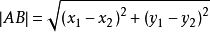Judge whether the distance between two centers is less than the sum of two radii:

``````Math.sqrt(
Math.pow(circleA.x - circleB.x, 2) + Math.pow(circleA.y - circleB.y, 2)
``````

Legend: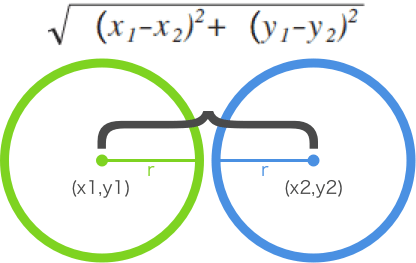Online operation example:

See the Pen EZrorG by Jc (@JChehe) on CodePen.

• Similar to "axisymmetric bounding box"

Applicable cases:

• (class) a circular object, such as a ball.

### Circle and rectangle (no rotation)

Concept: find out the closest point on the rectangle to the center of the circle, and then judge whether the distance between the point and the center of the circle is less than the radius of the circle. If it is less than, it is a collision.

How to find the nearest point on the rectangle to the center of the circle? Next, we look for the x-axis and y-axis respectively. To facilitate the description, we first agree on the following variables:

`````` The closest point on the rectangle to the center of the circle is the variable: closestpoint = {x, y};
rect = {x, y, W, H}; / / top left corner and width height
circle = {x, y, R}; / / center and radius
``````

First, the x-axis:

If the center of the circle is to the left of the rectangle `（if(circle.x < rect.x)）`
that `closestPoint.x= rect.x`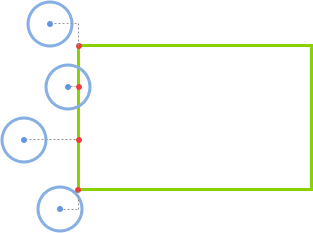If the center of the circle is to the right of the rectangle `(else if (circle.x > rect.x + rect.w))`
that `closestPoint.x = rect.x + rect.w`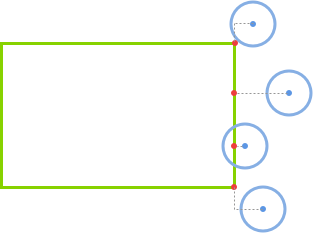If the center of the circle is directly above and below the rectangle `(else)`
that `closestPoint.x = circle.x`.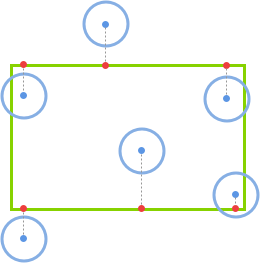Similarly, for the y-axis (not illustrated here):

If (circle. Y < rect. Y)`that`closestPoint.y = rect.y`.

If the center of the circle is below the rectangle `(else if (circle.y > rect.y + rect.h))`
that `closestPoint y = rect.y + rect h`.

The center of the circle is on the right and left sides of the rectangle `(else)`
that `closestPoint.y = circle.y`.

Therefore, the nearest point on the rectangle to the center of the circle can be found by the above method, and then the distance between the nearest point and the center of the circle can be obtained by the distance formula between the two points. Finally, the collision can be judged by comparing it with the radius of the circle.

``````var distance = Math.sqrt(Math.pow(closestPoint.x - circle.x, 2) + Math.pow(closestPoint.y - circle.y, 2))

if(distance < circle.r) return true // collide
Else return false / / no collision occurred
``````

Online operation example:

See the Pen Circle and Rectangle by Jc (@JChehe) on CodePen.

-The rectangle must be axisymmetric, that is, it cannot be rotated.

### Circle and rotation rectangle (with the center of the rectangle as the rotation axis)

Concept: even if the rectangle rotates with its center as its rotation axis, the essence of judging whether it collides with the circle is to find the nearest point on the rectangle from the center of the circle.

For the rotated rectangle, it is difficult to find the closest point to the center of the circle. In fact, we can expand the scope of our thinking: the rotation of the rectangle is regarded as the rotation of the whole canvas. So when we rotate the canvas (i.e. canvas) in the opposite direction, the result is the situation of the previous method "circle and rectangle (no rotation)". Therefore, we only need to figure out the center position of the canvas after rotation, and then we can use the judgment method of "circle and rectangle (no rotation)".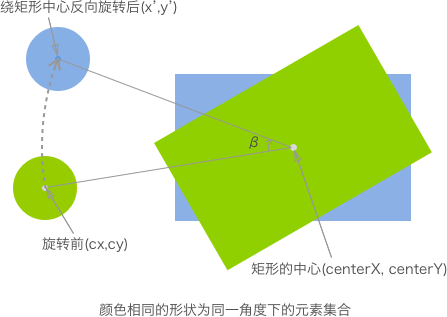First, the formula that can be applied directly is given, so as to obtain the center coordinates after rotation:

``````x’ = cos(β) * (cx – centerX) – sin(β) * (cy – centerY) + centerX
y’ = sin(β) * (cx – centerX) + cos(β) * (cy – centerY) + centerY
``````

It can be concluded from the above formula that the coordinate (C, d) after rotation is only related to the coordinate (x, y) before rotation and the rotation angle β.

Of course, (C, d) is the coordinate relative to the rotation point (axis) after rotating a certain angle. Therefore, the coordinate value of the center point of the rectangle is added to the "directly applicable formula" mentioned above.

From the figure, we can also draw the following conclusion: point C after point a rotates always moves on the circumference (radius is | ab |), using this point, we can make the object move around the rotation point (axis) in a circle.

After getting the coordinate value of the center of the circle after rotation, you can use the "circle and rectangle (no rotation)" method for collision detection.

Online operation case:

See the Pen Circle and Rotated Rectangle Collision Detection by Jc (@JChehe) on CodePen.

• Compared with the method of circle and rectangle (not rotating), it has a wider application range.

## Others

### Map grid division

Concept: divide the map (scene) into grids. In the map, all the objects involved in the detection store the coordinates of their own lattice, so you can think that two objects are collision when they are adjacent to each other, or two objects are collision only when they are in the same lattice. In addition, the premise of using this method is that all possible collision objects in the map should be the size of the lattice unit or an integral multiple of it.

Blue x is the obstacle: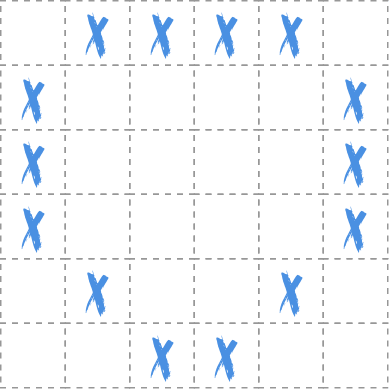Implementation method:

``````// Designated (non) feasible area by specific identification
map = [
[0, 0, 1, 1, 1, 0, 0, 0, 0],
[0, 1, 1, 0, 0, 1, 0, 0, 0],
[0, 1, 0, 0, 0, 0, 1, 0, 0],
[0, 1, 0, 0, 0, 0, 1, 0, 0],
[0, 1, 1, 1, 1, 1, 1, 0, 0]
],
// Set the initial position of the character
player = {left: 2, top: 2}

// Judge the next action of the character before (after) moving (if unable to move forward)
...
``````

Online operation example:

See the Pen map cell collision detection by Jc (@JChehe) on CodePen.

• Application scenario limitations.

Applicable cases:

• Pushing boxes, stepping on mines, etc

### Pixel detection

Concept: use pixel level to detect whether there is overlap between objects, so as to judge whether there is collision.

There are many ways to implement it. Here are two ways to implement it in canvas:

1. In the following case, it is determined whether there are non transparent pixels under the same position (coordinate) by putting two objects in the off screen canvas.

2. Use canvas's globalcompositeoperation = 'destination-in' attribute. This attribute keeps the overlap of the two and makes the rest transparent. Therefore, if there are non transparent pixels, they are collisions.

Note that when there are two collision objects to be detected, the first method requires two off screen canvass, while the second method requires only one.

Off screen canvas: related to it is off screen rendering. As its name suggests, it renders somewhere, but not on the screen. "Somewhere" is actually memory. Rendering to memory is faster than rendering to screen. —— Offscreen Rendering

Of course, we do not take advantage of the performance advantages of the off screen render, but use the off screen canvas to save the pixels of independent objects. In other words: onscreen canvas is only for display, and collision detection is carried out in offscreen canvas.

In addition, due to the need of pixel by pixel detection, if all the pixels in the entire canvas are operated, it will undoubtedly waste a lot of resources. Therefore, we can get the intersecting region by operation first, and then only detect the pixels in the region.

Legend: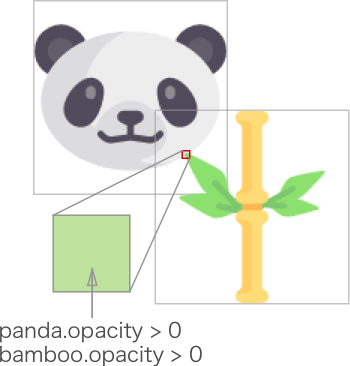The following example shows the first implementation:

See the Pen pixel collision detection by Jc (@JChehe) on CodePen.

• Because it is necessary to check every pixel to determine whether it collides, the performance requirements are relatively high.

Applicable cases:

• It needs to detect whether objects collide at pixel level.

## Ray casting

Concept: by detecting whether the velocity vectors of two objects have an intersection, and the intersection meets certain conditions.

For the following case of throwing a small ball into the barrel: draw a line (ා1) that coincides with the speed vector of the object, then start from another object to be detected, connect to the previous object, draw the second line (ා2), and determine whether the collision occurs according to the intersection position of the two lines.

Legend of throwing the ball into the barrel: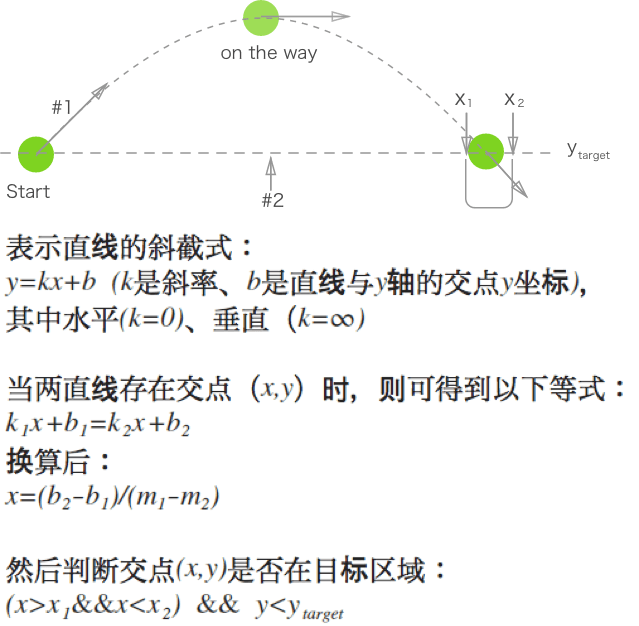In the process of small ball flying, the intersection of two straight lines needs to be calculated continuously.

When the following two conditions are met, the application can determine that the ball has fallen into the bucket:

-The intersection of the two lines is between the left and right edges of the barrel mouth
-The ball is below the second line (ා2)

Online operation example:

See the Pen ray casting collision detection by Jc (@JChehe) on CodePen.

-Suitable for fast moving objects

• The scope of application is relatively limited.

Applicable cases:

• Throw the ball into the barrel.

## Separating axis theorem

Concept: judge whether the projection of any two convex polygons at any angle overlaps to determine whether there is collision. If there is a gap between the projections of two objects under a certain angle light source, it is non-collision, otherwise it is collision.

Legend: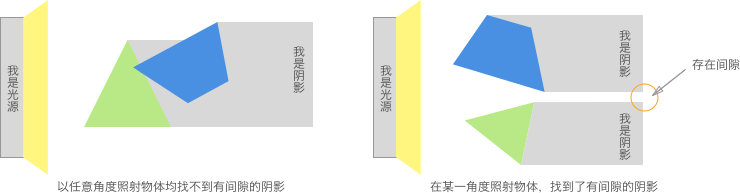In a program, it's unrealistic to traverse all angles. How to determine the projection axis? In fact, the number of projection axis is equal to the number of sides of the polygon.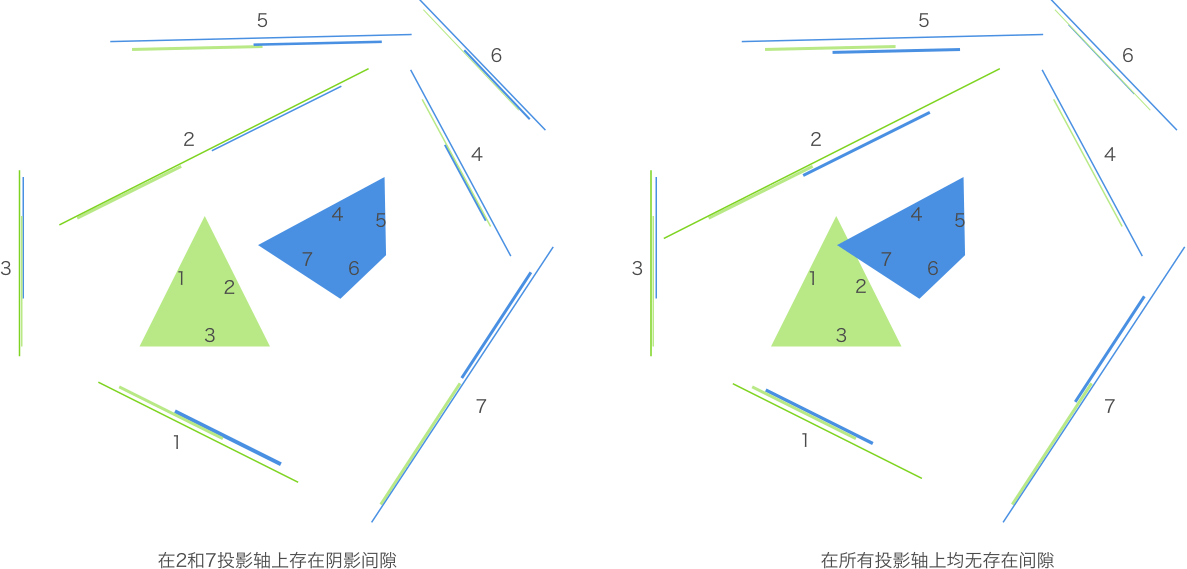To judge whether two convex polygons collide at a higher level of abstraction:

``````function polygonsCollide(polygon1, polygon2) {
var axes, projection1, projection2

//Get all projection axes from polygons
axes = polygon1.getAxes()
axes.push(polygon2.getAxes())

//Traverse all projection axes to obtain the projection of polygons on each projection axis
for(each axis in axes) {
projection1 = polygon1.project(axis)
projection2 = polygon2.project(axis)

//Judge whether the projection on the projection axis overlaps or not. If a gap is detected, immediately exit the judgment and eliminate unnecessary operations.
if(!projection1.overlaps(projection2))
return false
}
return true
}
``````

There are several points to be solved in the above code:

• How to determine the projection axis of a polygon
• How to project a polygon onto a projection axis
• How to detect whether two projections overlap

### Projection axis

As shown in the figure below, we use a vector from P1 to P2 to represent an edge of a polygon, which we call an edge vector. In the separation axis theorem, we also need to determine a normal vector perpendicular to the edge vector, which we call "edge normal vector".

The projection axis is parallel to the edge normal vector. The position of projection axis is unlimited, because its length is infinite, so the projection of polygon on this axis is the same. The direction of the axis is the key.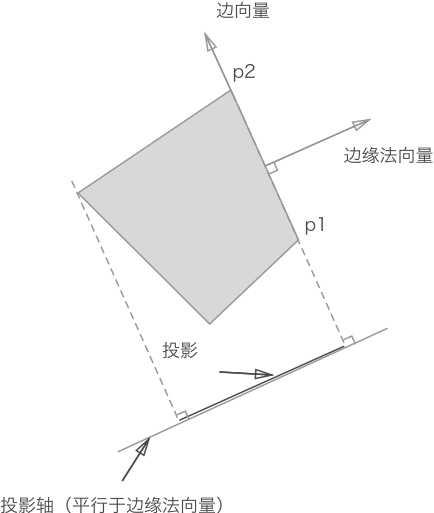``````// Start with the origin (0,0) and end with the vertex. Finally, the edge vector is obtained by vector subtraction.
var v1 = new Vector(p1.x, p1.y)
v2 = new Vector(p2.x, p2.y)

// First, the edge vector is obtained, and then the corresponding edge normal vector (unit vector) is obtained through the edge vector.
// The two vectors are subtracted to get the edge vector p2p1 (Note: there should be a right arrow above to represent the vector).
// Let the vector p2p1 be (a, b), then its normal vector can be obtained by x1x2 + y1y2 = 0: (- B, a) or (B, - a).
axis = v1.edge(v2).normal()
``````

The following is a partial implementation of the vector object. See the source code for details.

``````var Vector = function(x, y) {
this.x = x
this.y = y
}

Vector.prototype = {
// Obtain the vector size (i.e. the module of the vector), i.e. the distance between two points
getMagnitude: function() {
return Math.sqrt(Math.pow(this.x, 2),
Math.pow(this.y, 2))
},
// One of the geometric meanings of point product is the numerical product of the projection of one vector in the direction parallel to another vector.
// It will be used later to calculate the projection length
dotProduct: function(vector) {
return this.x * vector.x + this.y + vector.y
},
// Vector subtraction to get edge
subtarct: function(vector) {
var v = new Vector()
v.x = this.x - vector.x
v.y = this.y - vector.y
return v
},
edge: function(vector) {
return this.substract(vector)
},
// Get the normal vector of the current vector (vertical)
perpendicular: function() {
var v = new Vector()
v.x = this.y
v.y = 0 - this.x
return v
},
// Obtain the unit vector (that is, the vector size is 1, which is used to represent the vector direction). Divide a non-zero vector by its module to get the unit vector
normalize: function() {
var v = new Vector(0, 0)
m = this.getMagnitude()
if(m !== 0) {
v.x = this.x / m
v.y = this.y /m
}
return v
},
// Obtain the unit vector of edge normal vector, i.e. projection axis
normal: function() {
var p = this.perpendicular()
return p .normalize()
}
}
``````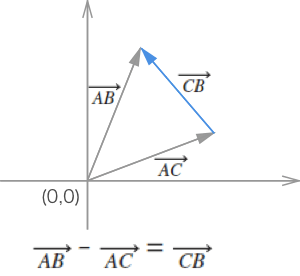Vector subtraction

More knowledge about vectors can be learned through other channels.

Projection size: by projecting each vertex of a polygon on a certain projection axis with a vector composed of the origin (0,0), and then keeping the maximum and minimum values of all projections of the polygon on the projection axis, the projection of a polygon on a certain projection axis can be represented.

To judge whether the projections of two polygons coincide: `projection1.max > projection2.min & & project2.max > project.min`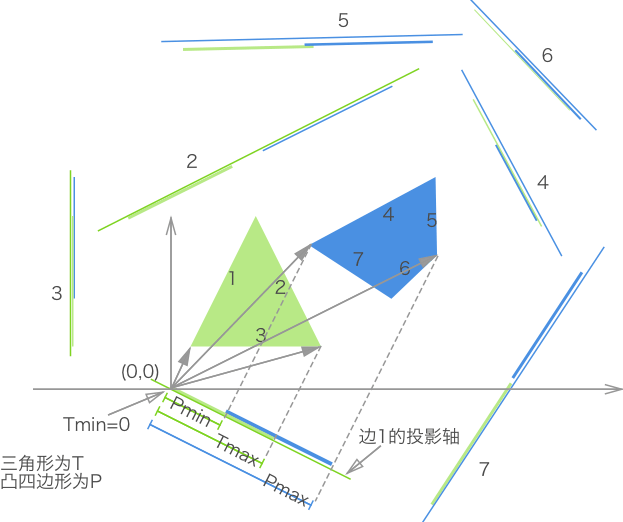For ease of understanding, the example graph places the axis origin (0,0) at the appropriate position of the projection axis of triangle edge 1.

The projection object can be obtained from the above:

``````//The projection position of a convex polygon on a projection axis is represented by the maximum and minimum values
var Projection = function (min, max) {
this.min
this.max
}

projection.prototype = {
//Judge whether two projections overlap
overlaps: function(projection) {
return this.max > projection.min && projection.max > this.min
}
}
``````

How to get the length of vector on projection axis?
One of the geometric meanings of the dot product of a vector is the numerical product of the projection of a vector in the direction parallel to another vector.
Since the projection axis is a unit vector (length 1), the projection length is X1 * x2 + Y1 * Y2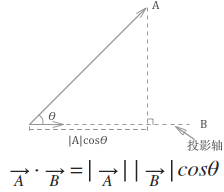``````// According to each point of the polygon, the maximum and minimum projection values are obtained to represent the projection.
function project = function (axis) {
var scalars = [], v = new Vector()

this.points.forEach(function (point) {
v.x = point.x
v.y = point.y
scalars.push(v.dotProduct(axis))
})
return new Projection(Math.min.apply(Math, scalars),
Math.max,apply(Math, scalars))
}
``````

### Collision detection between circle and polygon

Because the circle can be approximately regarded as a regular polygon with numerous edges, it is impossible for us to project and test one by one according to these edges. We only need to project the circle onto a projection axis, which is the line between the center of the circle and the nearest point in the polygon vertex, as shown in the figure: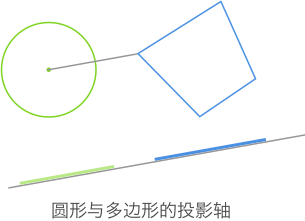Therefore, the projection axis and the projection axis of the polygon form a group of projection axes to be detected.

However, the collision detection between circles is still whether the distance between the original two centers is less than the sum of the two radii.

For the overall code implementation of the separation axis theorem, see the following cases:

See the Pen SeparatingAxisTheorem by Jc (@JChehe) on CodePen.

• precision

• Not for concave polygons

Applicable cases:

• Arbitrary convex polygons and circles.

### Extend: minimum translation vector (MIT)

Generally speaking, if the two sides still exist after the collision, then they need to be separated. After separation, the two objects that collided with each other can be bounced away, they can also be glued together, and other behaviors can be realized according to specific needs. But the first thing to do is to separate the two, which requires the minimum translation vector (MIT).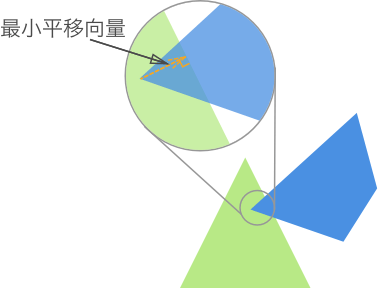## Collision performance optimization

If we need to judge all objects in two times in each period, it will cause waste (because some objects are distributed in different areas, and will not collide at all). Therefore, most games will divide the collision into two stages: coarse and fine (broad / narrow).

Broad phase can provide you with a list of possible collision entities. This can be achieved by special data structures that provide you with information about where entities exist and what entities are around them. These data structures can be Quad trees, R-trees or spatial HashMap.

If readers are interested, they can consult relevant information by themselves.

### Narrow phase

When you have a smaller list of entities, you can use the fine-grained algorithm (such as the collision algorithm described above) to get an exact answer (whether there is a collision).

## Last minute

There are many kinds of collision detection, and choosing the right one is the most important.

Reprinted to:“等一下，我碰！”——常见的2D碰撞检测